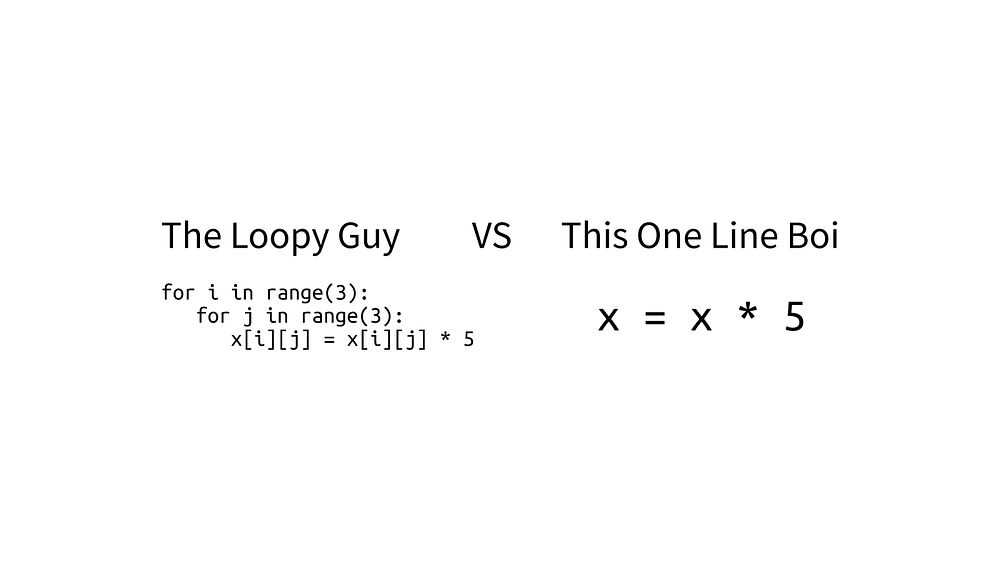# Matrix Computation With NumPy

Original article was published on Deep Learning on Medium# Introduction

Performance is really an important aspect when we are solving problems using Machine Learning, especially Deep Learning. Using a neural network for calculating things can become a complex calculation because it involves matrix and vectors to it.

Suppose we want to calculate a 25 x 25 matrix that is being multiplied by 5. At the first time you are being introduced to programming, You will calculate vectors or matrices that look like this,

`for i in range(25): for j in range(25): x[i][j] *= 5`

If we use this method, it will make our computation longer and we will not get the result at the time that we desired. Thankfully, in Python, we have NumPy library to solve this matrix and vector calculation.

By using NumPy library, we can make that 3-lines of code become 1-line of code like this one below,

`import numpy as np# Make it as NumPy array firstx = np.array(x)x = x * 5`

And if we compare the time, for this case, the conventional way will take around 0.000224 and the NumPy method is just 0.000076. The NumPy is almost 3 times faster than the conventional one, but it also simplifies your code at the same time!

Just imagine when you want to calculate a bigger matrix than in this example here and imagine how much the time that will you save. This calculation is classified as vectorization where the calculation has occurred on a matrix or vector representation. Therefore, with that computation method, it will save your time for the same result.

How is that happen? What is the magic of NumPy so it can calculate the matrix simpler and faster? This article will introduce you to the concepts of Single Instruction Multiple Data (SIMD) and Broadcasting. Without further, let’s get to it.